# 评估分形指数和Hurst指数预测金融时间序列的能力

15 七月 2019, 08:37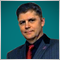0
1 412

### 分形维数的概念与时间序列的统计性质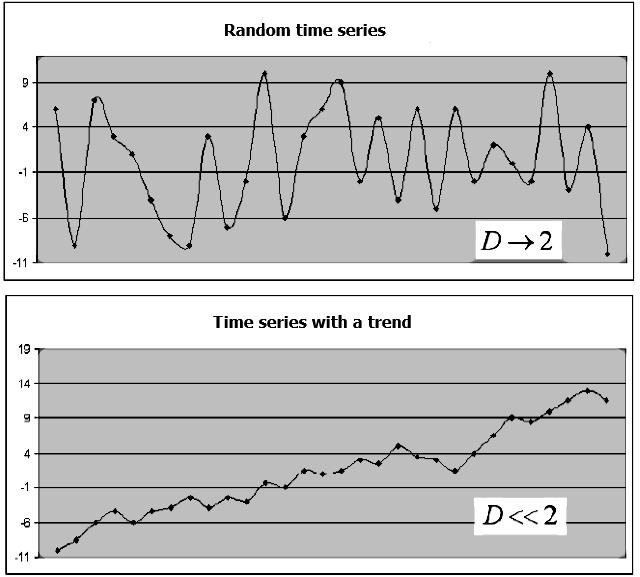### 分形维数评价方法及其特征

Hurst 指数 H 根据以下方程式确定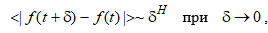(1a)

DH = 2-H
H = log(R/ S) / log(N / 2) (1b)

### 基于最小覆盖面积的分形维数估计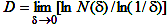.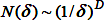(2)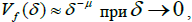(3)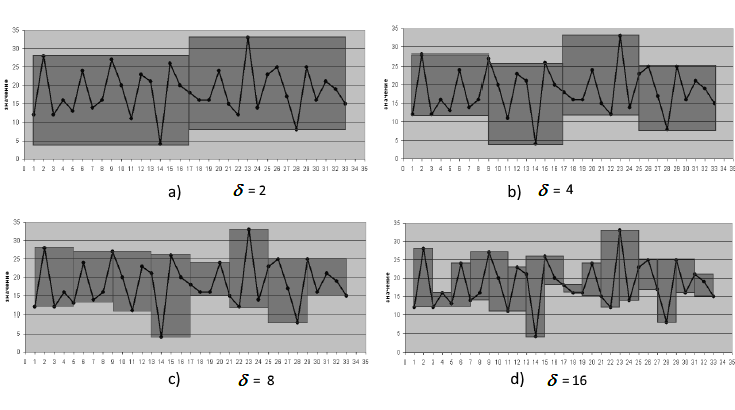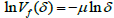(4)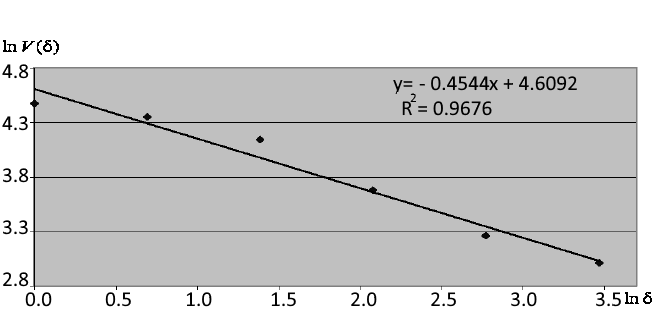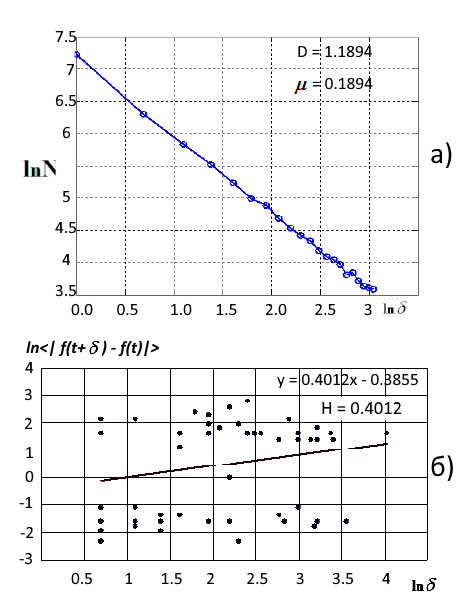• H — Hurst exponent;
•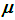— 分形指数;
•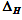H的置信区间宽度95%
•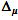置信区间的95%宽度
•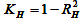- H的实验和获得的直线对应的准确度；
•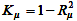- 实验结果与得到的直线符合度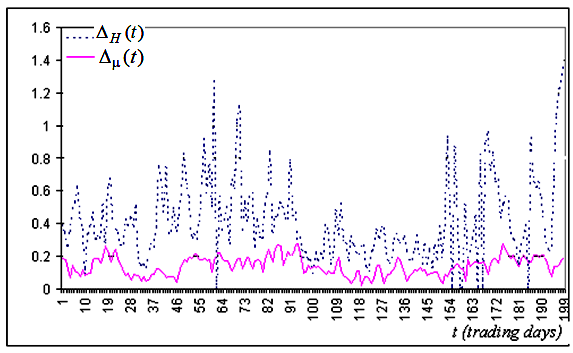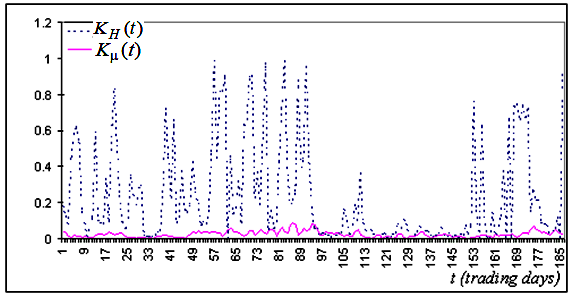### 时间序列性质与分形指数的相关性

1.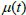= 0.5 表示随机价格波动（维纳过程）投资者行为独立，价格走势不明显。在这种情况下，我们可以说价格具有“正常”的稳定性，因为价格对外部影响的依赖性较弱，没有“反馈”，因此没有套利机会。
2.< 0.5 表明该价格对外部影响具有较高的稳定性，这与投资者对相关公司稳定性的信心以及市场上没有任何新信息有关。在这种情况下，股票价格在相当窄的价格范围内波动。当价格上涨时仍有足够的卖家，当价格下跌时也有足够的买家，他们的行为使价格回到初始范围。在这种情况下，“相关性”是负的，它在保持稳定的价格行为的同时减轻了股票价格的变化。
3.> 0.5 对应于降低的价格稳定性。这可能表明新信息的出现和对此信息的反应。可以假设，所有市场参与者对输入信息的估计大致相等，因此，与所收到的信息相对应的价格变动出现了趋势。某些情况下，这种情况会导致股票价格的急剧变化。

1.= 0.5, H = 0.5 时间序列是维纳过程（“布朗”噪声）。这个过程的主要特性是没有内存：序列演化与以前的值没有联系。
2. 当 0.5 <<= 1, 0 <= H < 0.5, 这个过程被认为是“粉红色”的噪音。它的特点是“负”记忆：如果过去记录的是正增量，它可能后面跟着负增量，反之亦然。
3. 如果 0 <=< 0.5 , 0.5 < H <=1, 时间序列是一种带有积极记忆的“黑色”噪声：如果过去发生了积极的趋势，它很可能会在未来继续存在，反之亦然。

### 分形指数和 Hurst 指数的评价指标

```double CFractalIndexLine::CalculateFractalIndex(const double &series[],const int N0,const int N1,
const double hourSampling,int CountFragmentScale=0)
{
//- - - - - - - - - - - - - - - - - - - - - - - - - - - - - - - - - - - - - - - - - - - - - - - - - - - - - - - - - - - - - - - - -
// series[] - time series
// N0, N1 - the left and right boundary points of the series[] array fragment, based on which the fractal index will be estimated
// hourSampling - discretization between points in HOURS
// CountFragmentScale - the number of requested scales to form a set of points, for which the fractal index is calculated
//
// RESULT
// the fractal index (Mu), the Hurst index (Hurst), the Confidence interval 95% (ConfInterval,
// coefficient of determination (R2det) - the closer to 1, the more accurately the calculation points fall on the approximating line
// determining stability for coefficient KR2 = 1-R2det. The closer to zero, the more accurate the calculated value of Mu
//- - - - - - - - - - - - - - - - - - - - - - - - - - - - - - - - - - - - - - - - - - - - - - - - - - - - - - - - - - - - - - - - -
// 1. Load the internal fragment with values from the time series
LoadFragment(series,N0,N1,hourSampling);

// 2. Determine the number of cycles to determine the points of the approximating line
int   nn2 = (int)floor(Nfrgm/2);  // Partition limits - no less than two points
int npow2 = (int)ipow2(nn2);      // The number of the powers of two in the Possible partitioning limit;

if(CountFragmentScale==0) CountFragmentScale=npow2; // default

int Count=fmin(CountFragmentScale,npow2);           // limiting the number of variants of series fragment division
int NumPartDivide;

for(int i=0; i<=Count; i++)
{
NumPartDivide = (int)pow (2,i);      // Number of pieces in the series fragment division
CalcAmplVariation(NumPartDivide, i); // Calculating a point for the approximating line model
i=i;
}
// 4. Evaluation of the Fractal Index and on the limits of the Index confidence intervals
Mu=fCalculateConfidenceIntervalMU(LogDeltaScales,LogAmplVariations,Count,ConfInterval,R2det);
Hurst=1-Mu;		// Hurst exponent
KR2=1-R2det;

return Mu;
}
//----------------------------------------------------------------------------------------------------------------------------------```
```double CFractalIndexLine::CalcAmplVariation(const int NumPartDivide,int idxAmplVar=-1)
{
// If idxAmplVar=-1, then index in the array is determined automatically (based on the contribution of the power of two in NumPartDivide)
// ALREADY PREVIOUSLY DONE: copying the fragment, setting the time of discretization of the series IN DAYS
// - - - - - - - - - - - - - - - - - - - - - - - - - - - - - - - - - - - - - - - - - - - - - - -

// 1. DETERMINE THE BORDERS OF INTERVALS CORRESPONDING TO THE SPECIFIC NUMBERS
int nCheckPoint=0,nIntervalPoints=0; // the number of points to check in one division interval
double dayDeltaScales=BoundaryArray(NumPartDivide,fragment,0,Nfrgm-1,hSampling,Boundaries,nIntervalPoints);

// 2. GO THROUGH INTERVALS TO DETERMINE LIMIT VALUES OF FUNCTIONS AND OF AMPLITUDE VARIATION
int countInterval=Boundaries.CountNonEmty();
int  maxFuncIdx=0,minFuncIdx=0;
double A,V=0.;

nCheckPoint=(int)(Boundaries.y-Boundaries.x)+1;
for(int i=0; i<countInterval; i++)
{
maxFuncIdx = ArrayMaximum(fragment,(int)Boundaries.x[i],nCheckPoint); // INDEX WITH MAX. VALUE
minFuncIdx = ArrayMinimum(fragment,(int)Boundaries.x[i],nCheckPoint);
A = fragment[maxFuncIdx] - fragment[minFuncIdx];
V = V+A;
i=i;
}

// 3. ACCUMULATION OF RESULTS IN STORAGE
if(idxAmplVar==-1) idxAmplVar=ipow2(NumPartDivide); // index in the storage array

LogDeltaScales   [idxAmplVar] = log(dayDeltaScales); // log-scale of the current division
LogAmplVariations[idxAmplVar] = log(V);              // log-Amplitude Variation in the current division scale

return V;
}
//--------------------------------------------------------------------------------------------------------------------------------------```

CFragmentIndexLine.mqh 文件片段执行覆盖面积计算循环，如图2所示。程序中的操作顺序通过详细的注释进行解释。

### 实际数据指标操作演示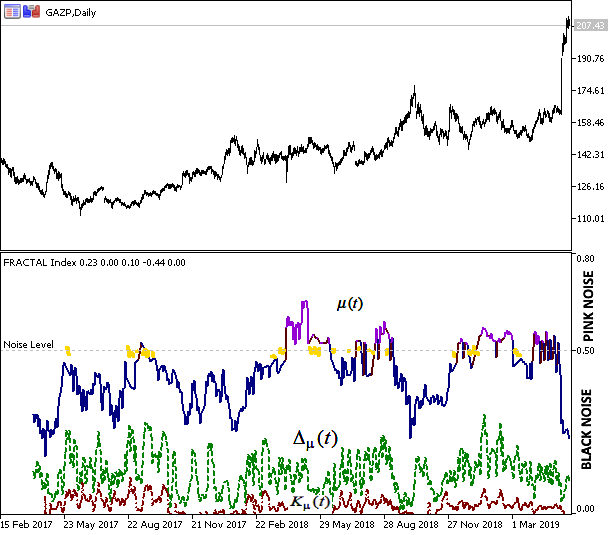### 结论

1. 确定无序状态，即时间序列的统计特征发生变化的时刻；
2. 时间序列的预测。

### 参考文献列表

1. Dubovikov M.M., Starchenko N.V. 金融资产时间序列的经济学与分形分析
2. Dubovikov M.M., Starchenko N.V. 经济学与金融时间序列分析"经济物理学. 现代物理学寻求经济理论”
3. Peters, J. 资本市场的混乱与秩序：周期、价格和市场波动的新视角
4. Krivonosova E.K., Pervadchuk V.P., Krivonosova E.A.经济指标时间序列的分形特征比较
5. Starchenko N.V. 物理应用中的局部分形分析。

FRACTAL_upd.zip (18.5 KB)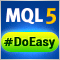轻松快捷开发 MetaTrader 程序的函数库(第七部分): StopLimit 订单激活事件，为修改订单和持仓事件准备功能轻松快捷开发 MetaTrader 程序的函数库(第六部分)：净持帐户事件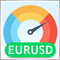价格速度测量方法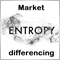通过差异化和熵值分析来探索市场"记忆"